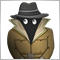How to calculate maximum value of 10 or more variables169

Hello,

I am saving values of a indicator in variable 10 times or more based on shift. Now I want to calculate the maximum and minimum value of those values. Whats the best way?

MathMax(v3,MathMax(v1,v2))..........?

Using arrays? I create array[] and resize and insert value from indicator? This option leaves only 1 time use of array and does not rewrite value into array.12328

Array Functions
 ArrayMaximum Search for an element with the maximal value ArrayMinimum Search for an element with the minimal value
Work with arrays. It will be easier.1502

Try CArrayDouble

```#include <Arrays/ArrayDouble.mqh>
//+------------------------------------------------------------------+
//|                                                                  |
//+------------------------------------------------------------------+
void OnStart()
{
CArrayDouble arr;

double max_value=arr.At(arr.Maximum(0,WHOLE_ARRAY));
}
```169

Konstantin Nikitin:
Array Functions
 ArrayMaximum Search for an element with the maximal value ArrayMinimum Search for an element with the minimal value
Work with arrays. It will be easier.

I followed the same thing, but once the array values are saved in array, it is not being over written in the next candle. how to calculate ArrayMaximum for new candle in EA? Resize array to 0 and again back to 10?169

amrali:

Try CArrayDouble

I never try this, will read and try it. will this Carraydouble overwrite values into array whenever the EA reads values from indicator?

I need a one dimension array, so can I give arr.Add(1)=SI(0,1) and it will be overwritten whenever the EA runs? SI value is taken from indicator every candle as below.

SI(int buff,int shift){ return(iCustom(NULL,PERIOD_H4,"tales",buff,shift));}749

Bhanu Sai Kishore Puvvala:

I followed the same thing, but once the array values are saved in array, it is not being over written in the next candle. how to calculate ArrayMaximum for new candle in EA? Resize array to 0 and again back to 10?

You can use a rotating index.

```static int idx=0;

arr[idx]=current_value;
idx=(idx+1) % 10;```

The index will increase by 1 for each iteration and reset to 0 if it reaches 10. So that new values always overwrite the eldest entry.12328

Bhanu Sai Kishore Puvvala:

I followed the same thing, but once the array values are saved in array, it is not being over written in the next candle. how to calculate ArrayMaximum for new candle in EA? Resize array to 0 and again back to 10?169

Konstantin Nikitin:

Can you give example of a code to use zeromemory and ArrayResize for 10 indexes to reset and write values to it on each cycle/candle?12328

Bhanu Sai Kishore Puvvala:

Can you give example of a code to use zeromemory and ArrayResize for 10 indexes to reset and write values to it on each cycle/candle?

Something like this...

```double test[], test_max, test_min;

bool Test(void)
{
ZeroMemory(test);
for(int i=0; i<10; i++)
{
if( ArrayResize(test, i+1) != i+1 )
return false;
test[i] = (iOpen(_Symbol, PERIOD_CURRENT, i) + iClose(_Symbol, PERIOD_CURRENT, i))/2;
}
test_max = test[ArrayMaximum(test,0)];
test_min = test[ArrayMinimum(test,0)];

return true;
}```